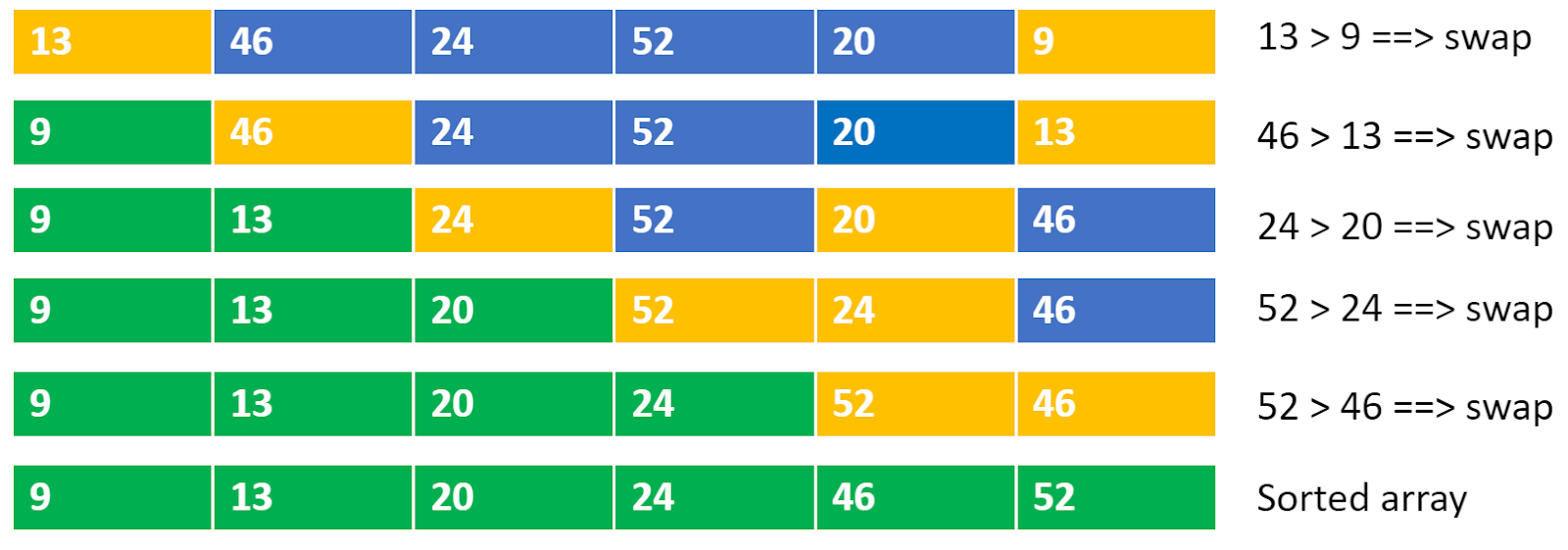# Selection Sort Algorithm

Problem Statement: Given an array of N integers, write a program to implement the Selection sorting algorithm.

Examples:

```Example 1:
Input: N = 6, array[] = {13,46,24,52,20,9}
Output: 9,13,20,24,46,52
Explanation: After sorting the array is: 9, 13, 20, 24, 46, 52

Example 2:
Input: N=5, array[] = {5,4,3,2,1}
Output: 1,2,3,4,5
Explanation: After sorting the array is: 1, 2, 3, 4, 5```

### Solution

Disclaimer: Don’t jump directly to the solution, try it out yourself first.

Solution 1:

Approach

• Find the minimum element in the unsorted array and swap it with the element at the beginning.
• The inner loop selects the minimum element in the unsorted array .
• In the below dry run,

Blue color represents unsorted array elements

The yellow color represents the elements to be swapped in an unsorted array

The green color represents the sorted array elementsCode:

## C++ Code

``````#include<bits/stdc++.h>

using namespace std;
void selection_sort(int arr[], int n) {
// selection sort
for (int i = 0; i < n - 1; i++) {
int mini = i;
for (int j = i + 1; j < n; j++) {
if (arr[j] < arr[mini]) {
mini = j;
}
}
int temp = arr[mini];
arr[mini] = arr[i];
arr[i] = temp;
}

cout << "After selection sort: " << "\n";
for (int i = 0; i < n; i++) {
cout << arr[i] << " ";
}
cout << "\n";

}
int main() {
int arr[] = {13,46,24,52,20,9};
int n = sizeof(arr) / sizeof(arr);
cout << "Before selection sort: " << "\n";
for (int i = 0; i < n; i++) {
cout << arr[i] << " ";
}
cout << "\n";
selection_sort(arr, n);
return 0;
}
``````

Output:

Before selection sort:
13 46 24 52 20 9
After selection sort:
9 13 20 24 46 52

Time Complexity: O(n^2) (nested for loops)

Space Complexity: O(1)

## Java Code

``````public class selection_sort {
public static void main(String args[]) {
int arr[] = {13,46,24,52,20,9};
int n = arr.length;
System.out.println("Before selection sort:");
for (int i = 0; i < n; i++) {
System.out.print(arr[i] + " ");
}
System.out.println();
//selection sort
for (int i = 0; i < n - 1; i++) {
int mini = i;
for (int j = i + 1; j < n; j++) {
if (arr[j] < arr[i]) {
mini = j;
}
}
//swap
int temp = arr[mini];
arr[mini] = arr[i];
arr[i] = temp;
}

System.out.println("After selection sort:");
for (int i = 0; i < n; i++) {
System.out.print(arr[i] + " ");
}
System.out.println();
}
}
``````

Output:

Before selection sort:
13 46 24 52 20 9
After selection sort:
9 13 20 24 46 52

Time Complexity: O(n^2) (nested for loops)

Space Complexity: O(1)

Special thanks to Rishiraj Girmal for contributing to this article on takeUforward. If you also wish to share your knowledge with the takeUforward fam, please check out this article